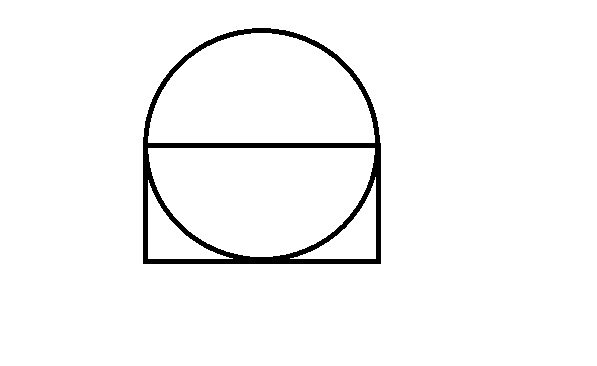## SBI Clerk DA Test 2

Instructions

Study the information given in each of these questions and then answer the question.

Question 1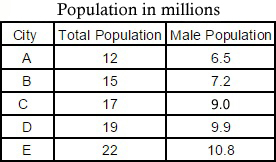What is the average female population in million?

Question 2

What is the percentage rise in production in 2007 from 2006? (Rounded off to two digits after decimal)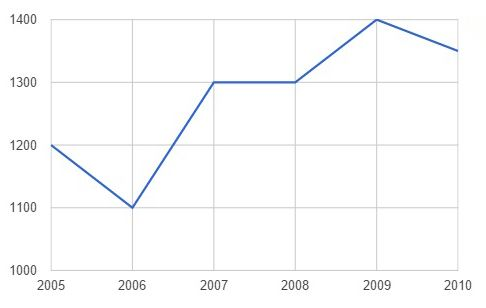Question 3

Out of the total 550 students, how many students did not prefer Maths or Economics?

Break-up of students having preference for each subject.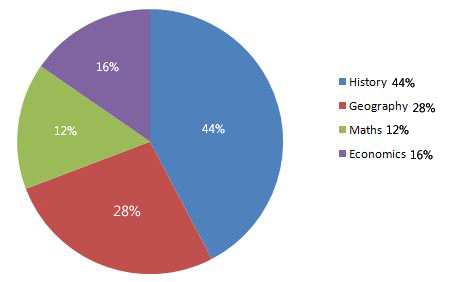Question 4

What is the difference (in Rs. lakhs) between the average salary and the lowest salary?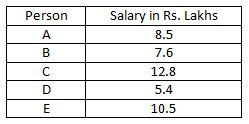Instructions

For the following questions answer them individually

Question 5

Area of the circle is 616 $$cm^{2}$$. What is the area of the rectangle? (One side of the rectangle passes through the centre of the circle)